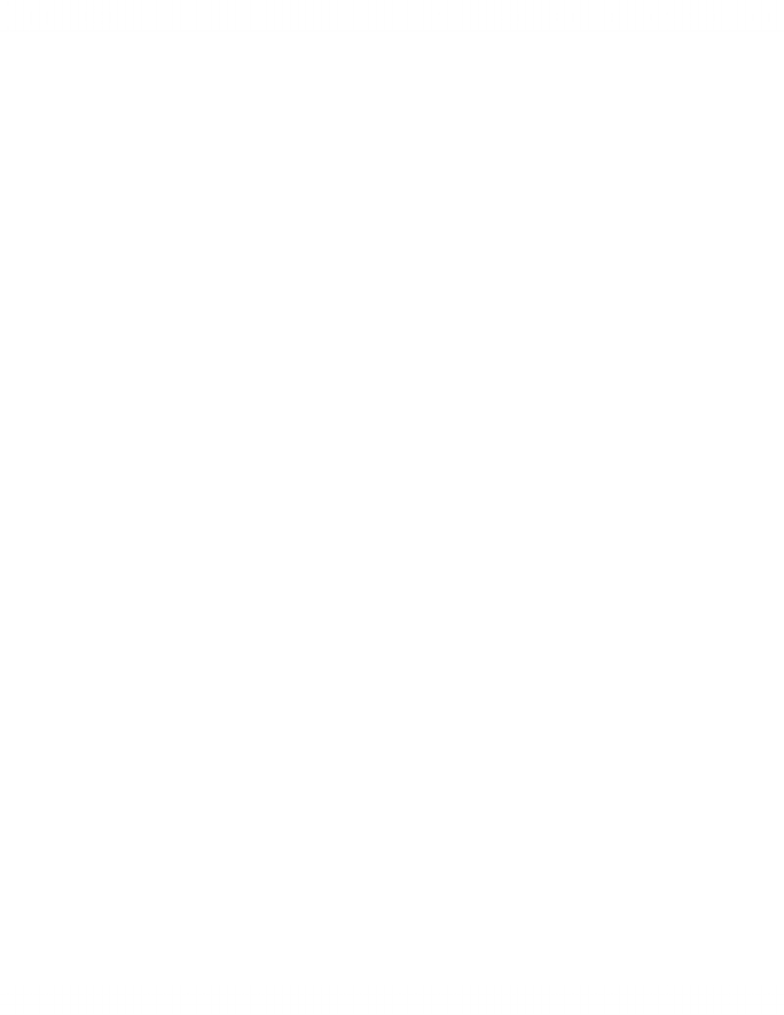Study Guides (380,000)
CA (150,000)
York (10,000)
Final

# ADMS 3530 Study Guide - Final Guide: Capital Structure, Credit Risk, Market Risk

Department
Course Code
Professor
Lois King
Study Guide
Final

This preview shows pages 1-3. to view the full 10 pages of the document.Practice Final Solutions
1. The followings are considered as financial assets, except:
A) well-trained sales force.
B) car insurance.
C) checking accounts.
D) mortgage loans.
A well-trained sales force is not a financial asset. All the others are
financial assets.
2. Which of the following does NOT address the question: "What are the roles of
a financial manager?"
I. Deciding how to maximize the stock prices.
II. Deciding the capital mix of long-term debt and equity.
III. Deciding which projects a firm should undertake.
IV. Deciding how much short-term debt to borrow.
A) I only
B) III only
C) II and III only
D) II, III, and IV only
E) I, II, III, and IV
Maximizing the stock price is not a role of the financial manager.
3. Given the following income statement data, calculate net income: sales =
\$2,500, cost of goods sold = \$1,800, miscellaneous expenses = \$200,
depreciation = \$150, interest expense = \$50, average tax rate = 35%.
A) \$195
B) \$230
C) \$377
D) \$425
EBT = \$2,500 - 1,800 - 200 - 150 - 50 = \$300; NI = \$300 - (300 x .35) =
\$195
1

Only pages 1-3 are available for preview. Some parts have been intentionally blurred.4. A firm with negative net working capital ______________.
A) is technically bankrupt
B) has no cash on hand
C) needs to sell some of its inventory to correct the problem
D) has more current liabilities than current assets
Net Working Capital = Current Assets – Current Liabilities
5. Calculate the EBIT (earnings before interest and taxes) for a firm with \$5
million total revenues, \$3 million cost of goods sold, \$500,000 depreciation
expense, and \$500,000 interest expense.
A) \$1,500,000
B) \$500,000
C) \$1,000,000
D) -\$500,000
EBIT = \$5,000,000 - \$3,000,000 - \$500,000 = \$1,500,000
6. What are the annual sales for a firm with \$400,000 in liabilities, a total debt
ratio of 0.25, and an asset turnover of 3.0 (assume total assets remains
unchanged)?
A) \$ 333,333
B) \$3,200,000
C) \$4,800,000
D) \$6,400,000
Total Debt Ratio = Total Liabilities / Total Assets = .25 = ¼
¼ = \$400,000/total assets, so total assets = \$1,600,000
Asset Turnover = Sales / Average Total Assets = 3.0
3.0 = Sales / \$1,600,000
So, Sales = \$4,800,000
7. How much will accumulate in an account with an initial deposit of \$100, and
which earns 15% interest for four years?
A) \$107.69
B) \$132.25
C) \$152.09
D) \$174.90
2

Only pages 1-3 are available for preview. Some parts have been intentionally blurred.\$100 x (1.15) ^4 = \$174.90
8. How much would an investor expect to pay for a \$1,000 par value bond with a
9% annual coupon that matures in 5 years if the interest rate is 5%?
A) \$ 844.4
B) \$1,075.8
C) \$1,082.0
D) \$1,173.2
PV = (90 x 4.3295) + (1000 x 0.7835) = \$1173.15
9. What is the coupon rate for a bond with three years until maturity, a price of
\$1,053.46, and a yield to maturity of 6%?
A) 6%
B) 8%
C) 10%
D) 11%
\$1053.46 = (PMT x 2.6730) + (1000 x 0.8396)
PMT = 80
Coupon Rate = 8%
10. What happens to the price of a three-year bond with an 8% coupon when
interest rates change from 8% to 6%?
A) A price increase of \$51.54
B) A price decrease of \$53.47
C) A price increase of \$53.47
D) No change in price
8%: (80 x 2.5771) + (1000 x 0.7938) = \$999.97
6%: (80 x 2.6730) + (1000 x 0.8396) = \$1053.44
Price increase of \$53.47
11. What is the yield to maturity of a bond with the following characteristics?
Coupon rate is 8% with annual payments, current price is \$950, three
years until maturity.
A) 4%
B) 5%
C) 10%
D) 12%
3
###### You're Reading a Preview

Unlock to view full version